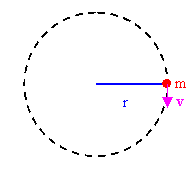Uniform circular motion

An object moving in a circle of radius r with constant speed v is accelerating.  The direction of its velocity vector is changing all the time, but the magnitude of the velocity vector stays constant.  The acceleration vector cannot have a component in the direction of the velocity vector, since such a component would cause a change in speed.  The acceleration vector must therefore be perpendicular to the velocity vector at any point on the circle.  This acceleration is called radial acceleration or centripetal acceleration, and it points towards the center of the circle.  The magnitude of the centripetal acceleration vector is ac = v2/r.  (We skip the derivation of this expression.)

Problem:

The orbit of the moon around the earth is approximately circular, with a mean radius of 3.85*108 m.  It takes 27.3 days for the moon to complete one revolution around the earth.  Find
(a) the mean orbital speed of the moon and
(b) its centripetal acceleration.

Solution:

• Reasoning:
The distance the moon travels in one orbital period T is d = 2πr.
Its speed is v = distance/time = d/T.
The centripetal acceleration of the moon is v2/r.
• Details of the calculation:
(a) The distance the moon travels in 27.3 days is d = 2πr = 2.41*109 m.
Its speed is v = d/(27.3 days) = (d/(2.36*106 s)) = 1023 m/s.
(b) The centripetal acceleration of the moon is v2/r = 2.725*10-3 m/s2.

An object moving in a circle, either with uniform or non-uniform speed, is accelerating.  Since it is accelerating, it must be acted on by a force.  Such a force is called a centripetal force.

Under different circumstances, what is the force?

Let us solve some problems investigating this question.

Problem:

3 kg mass attached to a light string rotates on a horizontal frictionless table.  The radius of the circle is 0.8 m and the string can support a mass of 25 kg before breaking.  What range of speeds can the mass have before the string breaks?Solution:

• Reasoning:
A mass attached to a string rotates on a horizontal, frictionless table.
We assume that the mass rotates with uniform speed.  It is accelerating.  The direction of the acceleration is towards the center of the circle, and its magnitude is v2/r.  There is tension in the string.  The string pulls on the mass with a force F directed towards the center of the circle.  This force F is responsible for the centripetal acceleration, F = mv2/r.
The string can support a mass of 25 kg before breaking, i.e. we can let a mass of up to 25 kg hang from the string near the surface of the earth.  The maximum tension in the string therefore is Fmax = mg = (25 kg)(9.8 m/s2) = 245 N.
Given Fmax = 245 N and F = mv2/r, we can find vmax.
• Details of the calculation:
vmax2 = Fmaxr/m = (250 N)(0.8 m)/(3kg).  vmax = 8.1 m/s.

Problem:

A coin placed 30 cm from the center of a rotating, horizontal turntable slips when its speed is 50 cm/s.
(a)  What force provides the centripetal acceleration when coin is stationary relative to the turntable?
(b)  What is the coefficient of static friction between coin and turntable?

Solution:

• Reasoning:
When the coin is at rest relative to the rotating turntable, the force of static friction between the coin and the turntable provides the centripetal acceleration.
The force of static friction has a maximum value fs = μsN = μsmg.
Setting μsmg = mv2/r, we can solve for μs.
• Details of the calculation:
(a)  The force of static friction between the coin and the turntable provides the centripetal acceleration.
(b)  The magnitude of the maximum force of static friction is fs = μsN.  This maximum force of static friction is equal to mv2/r when v = 0.5 m/s.  We have μsN = μsmg = mv2/r,
or μs = v2/(rg) = (0.5m/s)2/(0.3m 9.8m/s2) = 0.085.

Module 3: Question 1

Do you feel yourself thrown to either side when you negotiate a curve that is ideally banked for your car's speed? What is the direction of the force exerted on you by the car seat?

Discuss this with your fellow students in the discussion forum!